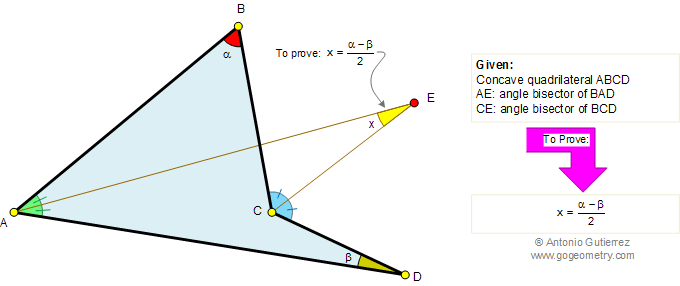Problem 383. Concave Quadrilateral, Angle Bisectors, Semi-difference of Angles. Level: High School, College, SAT Prep. The figure shows a concave quadrilateral ABCD with AE angle bisector of angle BAD and CE angle bisector of angle BCD. Prove that angle AEC = (angle ABC - angle ADC) / 2.Recent Additions